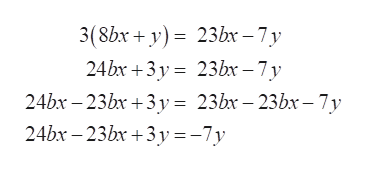# Hi, I need help solving the below problem are you able to provide each step as well? Thank youSolve for X.3(8bx+y)= 23bx-7y

Question

Hi, I need help solving the below problem are you able to provide each step as well? Thank you

Solve for X.

3(8bx+y)= 23bx-7y

check_circleExpert Solution
Step 1

Refer to the question , we have to calculate the value of x for the equation 3(8bx+y)= 23bx-7y

Step 2

To find the value of x try to isolate the variable x to one side and rest to other.

So here solve the left hand side of the equation by expanding it as,

Step 3

Now subtract 23bx to both sides of the equation to coll...help_outlineImage Transcriptionclose3(8bxy) 23bx-7y 24bx 3y 23b -7y 24bx 23bx 3y= 23bx 23bx- 7y 24bx 23bx +3y=-7y fullscreen

### Want to see the full answer?

See Solution

#### Want to see this answer and more?

Solutions are written by subject experts who are available 24/7. Questions are typically answered within 1 hour*

See Solution
*Response times may vary by subject and question
Tagged in

### Equations and In-equations